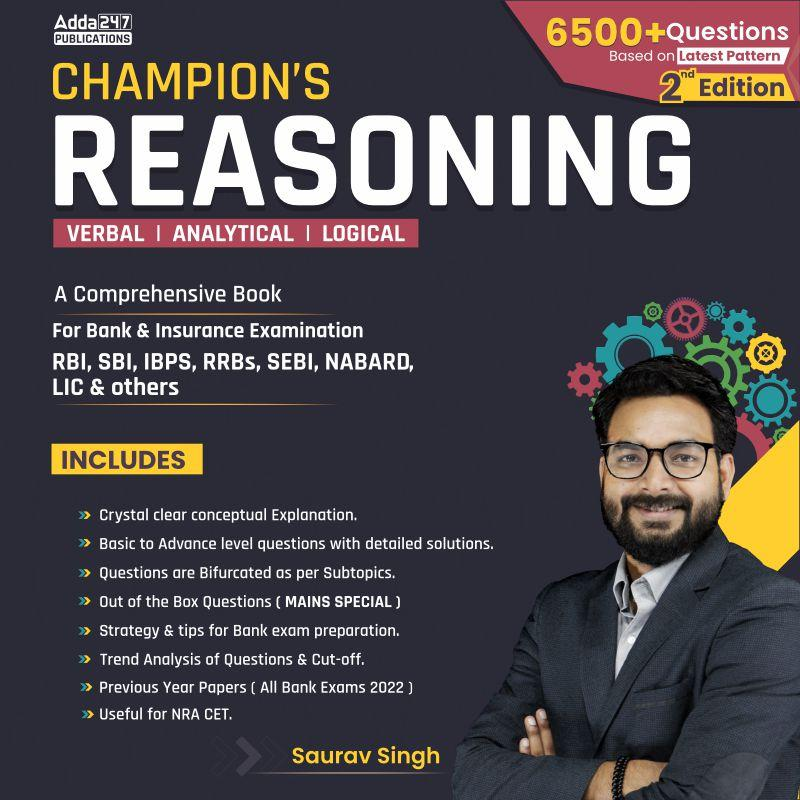Latest Banking jobs   »   IBPS reasoning   »   IBPS reasoning

# Reasoning Quiz For IBPS RRB PO/Clerk Mains 2023-02nd September

Directions (1-2): Answer the questions based on the information given below.
If'<‘ is denoted as ‘@’
‘>’ is denoted as ‘&’
‘=’ is denoted as ‘\$’
‘≤’ is denoted as ‘#’
And ‘≥’ is denoted as “%”
Note: The numbers are also taken as elements.

Q1. Statements: W@D%7&9; P@9; P\$K%F
Conclusions: I. W&F
II. K@D
(a) Only I is true
(b) Only II is true
(c) Either I or II is true
(d) Neither I nor II is true
(e) Both I and II are true

Q2. Statements: H&T% L\$V; G#W@V; G%D
Conclusions: I. H & D
II. T&G
(a) Only I is true
(b) Only II is true
(c) Either I or II is true
(d) Neither I nor II is true
(e) Both I and II are true

Direction (3-6): In the following questions assuming the given statement to be true, find which of the conclusion(s) among given conclusions is/are definitely true and then give your answers accordingly.

Q3. Statements: 8 ≥ Q < 6 ≤ F= X; Q > U; C=U
Conclusions: I. X > U
II. C < F
(a) Only I is true
(b) Only II is true
(c) Either I or II is true
(d) Neither I nor II is true
(e) Both I and II are true

Q4. Statements: M > N ≥ 6 = D >7; 6 > R > T < C; 7 > F = G < 9
Conclusions: I. T < M
II. G ≥ N
III. D > G
(a) Only I is true
(b) Only II is true
(c) Only I and II are true
(d) Only I and III is true
(e) None of these

Q5. Statements: X ≥ Q > T; M > Z ≥ Y > G; X < V < G
Conclusions: I. Z > Q
II. Y > T
III. V > M
(a) Only I is true
(b) Only II is true
(c) Only I and II are true
(d) Only I and III is true
(e) None of these

Q6. Statements: O < M=N; O > P; Q > C; H < C < P
Conclusions: I. H < N
II. C > O
III. P > Q
(a) Only I is true
(b) Only II is true
(c) Only I and II are true
(d) Only I and III is true
(e) None of these

Directions (7-8): Answer the questions based on the information given below.
If ‘<’ is denoted as ‘1’
‘>’ is denoted as ‘2’
‘=’is denoted as ‘3’
‘≤’ is denoted as ‘4’
And ‘≥’ is denoted as ‘5’

Q7. Statements: U1V5B2O; K1O; K3J5R
Conclusions: I. U2R
II. J1V
(a) Only I is true
(b) Only II is true
(c) Either I or II is true
(d) Neither I nor II is true
(e) Both I and II are true

Q8. Statements: H2M5B3X; P4S1X; P5D
Conclusions: I. H2D
II. M2P
(a) Only I is true
(b) Only II is true
(c) Either I or II is true
(d) Neither I nor II is true
(e) Both I and II are true

Directions (9-10): Answer the questions based on the information given below.
1*2 means 1 is not greater than 2.
1\$2 means 1 is not smaller than 2.
1#2 means 1 is neither greater than nor equal to 2.
1%2 means 1 is neither smaller than nor equal to 2.
1 @2 means 1 is neither greater than nor smaller than 2.

Q9. Statements: A\$T@N; S #O @Q* T; N%L
Conclusions: I. A% S
II. S % T
III. O \$ L
(a) Only I is true
(b) Only II is true
(c) Only I and II are true
(d) Only I and III is true
(e) None of these

Q10. Statements: P%G@L; N#G; L*H; J%P
Conclusions: I. J%L
II. H#N
III. P\$H
(a) Only I is true
(b) Only II is true
(c) Only I and II are true
(d) Only I and III is true
(e) None of these

Solutions

Solution (1-2):
S1. Ans. (b)
Sol. I. W&F – W >F – False
II. K@D – K < D – True

S2. Ans. (e)
Sol. I. H & D – H > D – True
II. T&G – T > G – True

Solution (3-6):
S3. Ans. (e)
Sol. I. X > U – True
II. C < F – True

S4. Ans. (d)
Sol. I. T < M – True
II. G ≥ N – False
III. D > G – True

S5. Ans. (c)
Sol. I. Z > Q – True
II. Y > T – True
III. V > M – False

S6. Ans. (a)
Sol. I. H < N – True
II. C > O – False
III. P > Q – False

Solution (7-8):
S7. Ans. (b)
Sol. I. U2R – U > R – False
II. J1V – J<V – True

S8. Ans. (e)
Sol. I. H2D – H>D – True
II. M2P – M>P – True

Solution (9-11):
S9. Ans. (a)
Sol. I. A% S – A>S – True
II. S % T – S>T – False
III. O \$ L – O≥L – False

S10. Ans. (a)
Sol. I. J%L –J>L – True
II. H#N – H<N – False
III. P\$H – P≥H – False## FAQs

### When will the IBPS RRB PO mains exam be conducted?

IBPS RRB PO mains exam will be conducted on 10 September 2023

#### Congratulations!Union Budget 2023-24: Free PDF